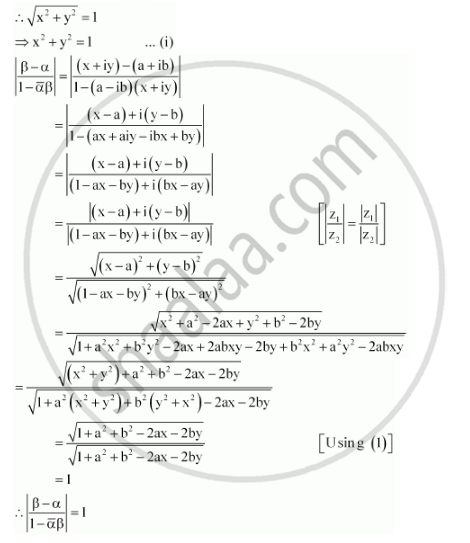CBSE (Arts) Class 11CBSE
Share

# If α and β Are Different Complex Numbers with |β| = 1, Then Find |(Beta - Alpha)/(1-baralphabeta)| - CBSE (Arts) Class 11 - Mathematics

ConceptAlgebra of Complex Numbers

#### Question

If α and β are different complex numbers with |β| = 1, then find |(beta - alpha)/(1-baralphabeta)|

#### Solution

Let α = a + ib and β = x + iy

It is given that, |β| = 1Is there an error in this question or solution?

#### Video TutorialsVIEW ALL 

Solution If α and β Are Different Complex Numbers with |β| = 1, Then Find |(Beta - Alpha)/(1-baralphabeta)| Concept: Algebra of Complex Numbers.
S